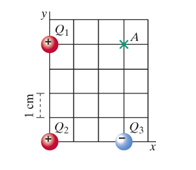+1-415-670-9189
info@expertsmind.com

# Get Solution

What is the electric field at the location
Course:- Physics
Reference No.:- EM13164

 TweetExpertsmind Rated 4.9 / 5 based on 47215 reviews.
Review Site
Assignment Help >> Physics

Field and force with three chargesAt a particular moment, three small charged balls, one negative and two positive, are located as shown in the figure. Q1 = 2 nC, Q2 = 6nC, and Q3 = -4 nC.

Given:

Q1 = 2 nC = 2*10-9 C

Q2 = 6 nC = 6*10-9 C

Q2 = -4 nC = -4*10-9 C

k = 8.988*109 N*m2/C2 is Coulomb's constant

(a1) What is the electric field at the location of Q1, due to Q2?
Ë2=   N/C

Minimize

Ask Question & Get Answers from Experts
Browse some more (Physics) Materials
 An Australian emu is running due east in a straight line at a speed of 17.6 m/s and slows down to a speed of 15.7 m/s in 7.7 s. Assuming that the acceleration remains the sa The power required by an ice maker is 225 W and is the rate at which it does work. The ice maker operates like a refrigerator or an air conditioner and has a coefficient of A dilute ideal gas of atoms each of mass M at temperature τ is placed in a cetrifuge rotating with angular velocity ω. Derive an expression for the relative density of gas mol The titanic was found in 1985 lying on the bottom of the north ocean.The pressure that the titanic felt at that depth was 400 times atmospheric pressure. what was the depth The density of seawater is 1025 kg/m3 and and density of ice is 917 kg/m3. Compute the weight of the heaviest bear that the ice can support without sinking completely beneath A 100 block on a frictionless table is firmly attached to one end of a spring with = 27 . The other end of the spring is anchored to the wall. A 22 ball is thrown horizontal A potato of mass 0.150 kg is tied to astring with length 2.10 m, and theother end of the string is tied to a rigid support. What is the tension in the string at this point An astronaut on the Mars attaches a small brass ball to a 1.00- length of string and makes a simple pendulum. She times 13 complete swings in a time of 35.4 seconds. From this